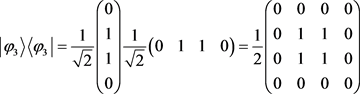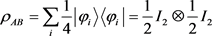﻿ 量子纠缠的相关性判据 The Correlation Criterion of QuantumEntanglement

Modern Physics
Vol. 09  No. 01 ( 2019 ), Article ID: 28329 , 4 pages
10.12677/MP.2019.91003

The Correlation Criterion of Quantum Entanglement

Guangrong Liu

Department of Foundations, Air Force Engineering University, Xi’an ShaanxiReceived: Dec. 12th, 2018; accepted: Dec. 27th, 2018; published: Jan. 2nd, 2019ABSTRACT

The separability judgment of quantum states is the basic problem of quantum entanglement theory. In this paper, based on Schmidt decomposition of two-body pure states, the correlation criteria of matrix rank and vector group are given. For the two-body mixed state, a sufficient and unnecessary condition for separability is given, and an example is given.

Keywords:Quantum Entanglement, Quantum State, Composite SystemCopyright © 2019 by author and Hans Publishers Inc.1. 引言

2. 量子纯态与复合系统

${|\phi 〉}_{AB}=\underset{i}{\sum }\underset{j}{\sum }{m}_{ij}|i〉〈j|$

3. 量子纯态纠缠

$\rho =|\phi 〉〈\phi |=\left({\xi }_{1},{\xi }_{2},\cdots ,{\xi }_{n}\right)$

4. 量子混合态纠缠

$\rho =\underset{i}{\sum }{p}_{i}|{\phi }_{i}〉〈{\phi }_{i}|$The Correlation Criterion of QuantumEntanglement[J]. 现代物理, 2019, 09(01): 19-22. https://doi.org/10.12677/MP.2019.91003

1. 1. Michael, A., Chuang, N.I.L., 赵千川. 量子计算与量子信息[M]. 北京: 清华大学出版社, 2004.

2. 2. Johnston, N., Kribs, D.W. and Teng, C.W. (2009) Operator Algebraic Formulation of the Stabilizer Formalism for Quantum Error Correction. Acta Applicandae Mathematicae, 108, 687-696.
https://doi.org/10.1007/s10440-008-9421-1

3. 3. Kribs, D.W., Laflamme, R., Poulin, D. and Lesosky, M. (2006) Operator Quantum Error Correction. Quantum Information and Computation, 6, 382-399.

4. 4. 张成杰. 量子纠缠的判定与度量[D]: [博士学位论文]. 合肥: 中国科学技术大学, 2010.

5. 5. 郑玉鳞. 量子纠缠与量子导引的判据研究[D]: [博士学位论文]. 合肥: 中国科学技术大学, 2016.